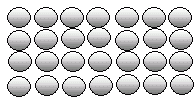Name: ___________________Date:___________________

 Email us to get an instant 20% discount on highly effective K-12 Math & English kwizNET Programs!

### Grade 2 - Mathematics7.9 Multiplication Review TestName: ___________________Date:___________________

### Grade 2 - Mathematics7.9 Multiplication Review Test

 Q 1: What is the multiplication form for: 2 + 2 + 2 + 2 + 2 + 22 x 5 = 102 x 2 = 42 x 6 =12 Q 2: Write the multiplication form for 6 + 6 + 6 + 66 + 6 + 6 + 6 = 246 x 5 = 306 x 4 = 24 Q 3: 4 x 7 =24282630 Q 4: 1 x 12 =120131 Q 5: What are the factors of the multiplication 76 x 4 = 30476 and 3044 and 30476 and 4 Q 6: Use the figure to find 4 times 7.252826 Q 7: Use the figure to find 4 times 6.232224 Q 8: 3 x 9 =28242527 Question 9: This question is available to subscribers only! Question 10: This question is available to subscribers only!